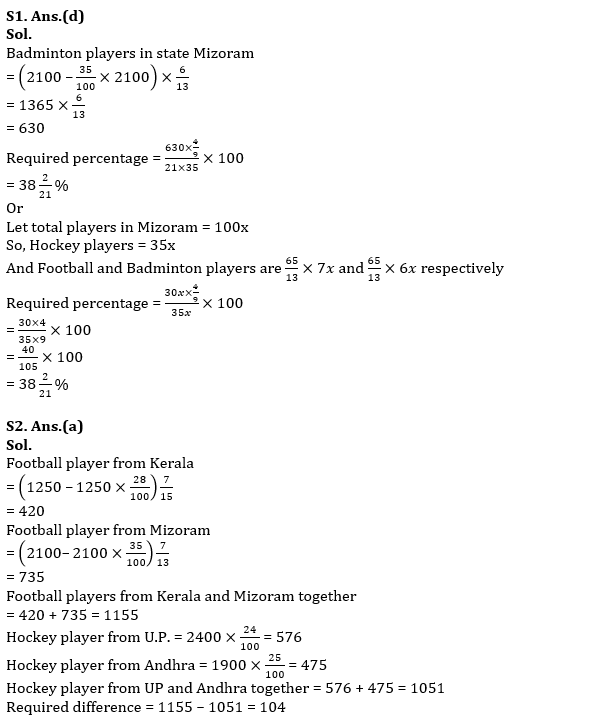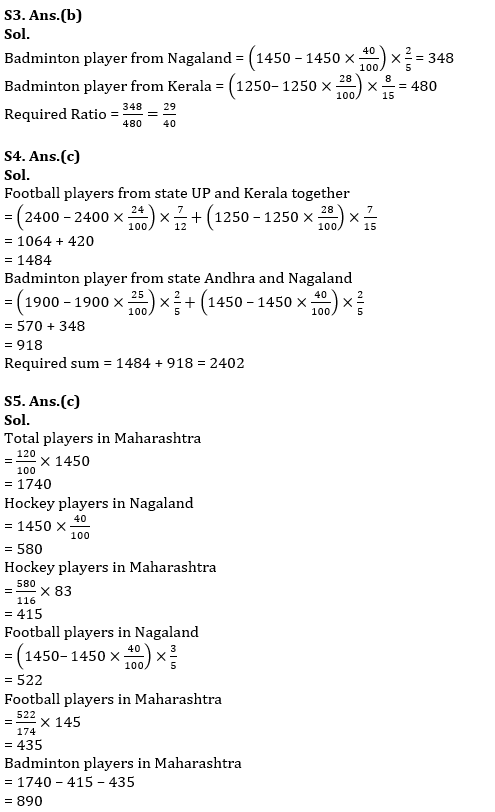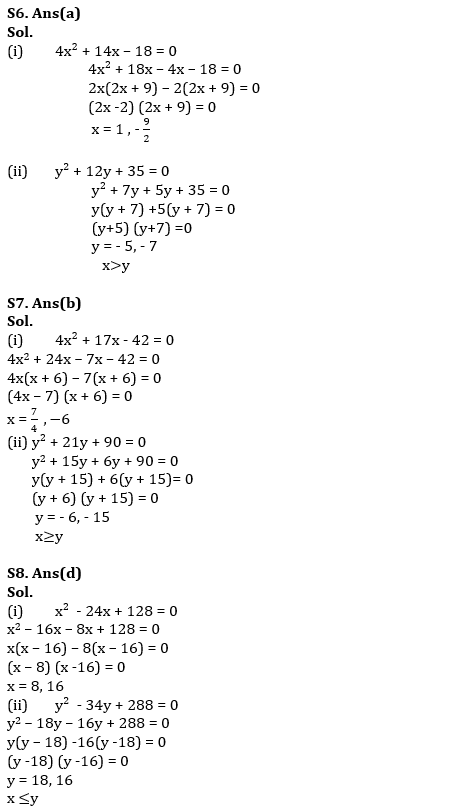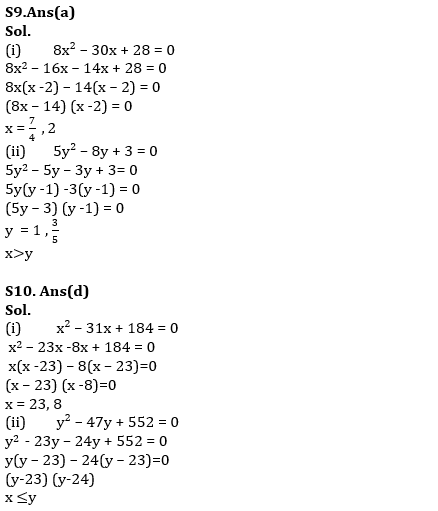Latest Banking jobs   »   Quantitative Aptitude Quiz For RBI Assistant/...

# Quantitative Aptitude Quiz For RBI Assistant/ ESIC UDC Mains 2022- 10th April

Directions (1–5): Table given below shows total players in five states and ratio of Football players to Badminton Players and the line graph shows percentage of Hockey players out of total players (Hockey, Football and Badminton). Read both table and line graph carefully and answer the questions: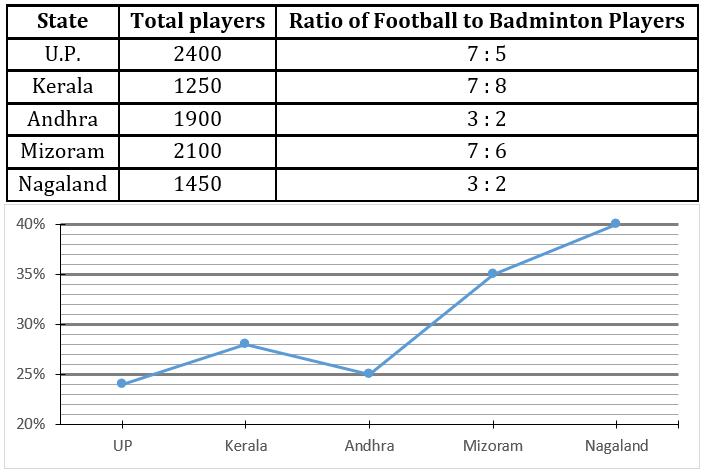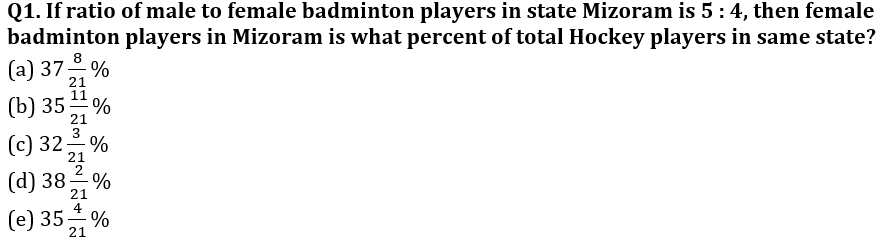Q2. Football players from state Kerala and Mizoram together is how many more or less than Hockey players from state UP and Andhra together?
(a) 104
(b) 91
(c) 95
(d) 110
(e) 93

Q3. Find the ratio between badminton players from Nagaland to badminton players from Kerala?
(a) 57 : 80
(b) 29 : 40
(c) 27 : 40
(d) 40 : 27
(e) 40 : 29

Q4. Find the total number of Football players from state UP and Kerala together and Badminton players from state Andhra and Nagaland together?
(a) 2627
(b) 2508
(c) 2402
(d) 3035
(e) 2480

Q5. In another state Maharashtra, total players who play these sports is 20% more than total players in Nagaland. If ratio of Hockey players and Football players in state Nagaland to state Maharashtra is 116 : 83 and 174 : 145 respectively then find total number of badminton players in Maharashtra?
(a) 850
(b) 870
(c) 890
(d) 910
(e) 930

Directions (6-10): In each of these questions, two equations are given. You have to solve both the equations and give answer

Q6. (i) 4x² + 14x – 18 = 0
(ii) y² + 12y + 35 = 0
(a) if x>y
(b) if x≥y
(c) if x<y
(d) if x ≤y
(e) if x = y or no relation can be established between x and y.

Q7. (i) 4x² + 17x – 42 = 0
(ii) y² + 21y + 90 = 0
(a) if x>y
(b) if x≥y
(c) if x<y
(d) if x ≤y
(e) if x = y or no relation can be established between x and y.

Q8. (i) x² – 24x + 128 = 0
(ii) y² – 34y + 288 = 0
(a) if x>y
(b) if x≥y
(c) if x<y
(d) if x ≤y
(e) if x = y or no relation can be established between x and y.

Q9. (i) 8x² – 30x + 28 = 0
(ii) 5y² – 8y + 3 = 0
(a) if x>y
(b) if x≥y
(c) if x<y
(d) if x ≤y
(e) if x = y or no relation can be established between x and y.

Q10. (i) x² – 31x + 184 = 0
(ii) y² – 47y + 552 = 0
(a) if x>y
(b) if x≥y
(c) if x<y
(d) if x ≤y
(e) if x = y or no relation can be established between x and y.

Solutions